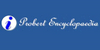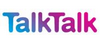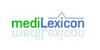### FactorialThe value of 0! is 1, according to the convention for an empty product. The factorial operation is encountered in many areas of mathematics, notably in combinatorics, algebra, and mathematical analysis. Its most basic occurrence is the fact that there are n! ways to arrange n distinct objects into a sequence (i.e., permutations of the set of objec...
Found on http://en.wikipedia.org/wiki/Factorial

### Factorial• (a.) Related to factorials. • (n.) A name given to the factors of a continued product when the former are derivable from one and the same function F(x) by successively imparting a constant increment or decrement h to the independent variable. Thus the product F(x).F(x + h).F(x + 2h) . . . F[x + (n-1)h] is called a factorial term, and it...
Found on http://thinkexist.com/dictionary/meaning/factorial/

### factorial1. Of or pertaining to a factory. ... 2. <mathematics> Related to factorials. ... <mathematics> A name given to the factors of a continued product when the former are derivable from one and the same function F(x) by successively imparting a constant increment or decrement h to the independent variable. Thus the product F(x).F(x + h).F(x...
Found on http://www.encyclo.co.uk/local/20973

### factorialadjective of or relating to factorials
Found on https://www.encyclo.co.uk/local/20974

### FactorialFac·to'ri·al adjective 1. Of or pertaining to a factory. Buchanan. 2. (Math.) Related to factorials.
Found on http://www.encyclo.co.uk/webster/F/2

### FactorialFactorial is a name in mathematics given to the factors of a continued product when the former are derivable from one and the same function F(x) by successively imparting a constant increment or decrement h to the independent variable. Thus the product F(x).F(x + h).F(x + 2h) . . . F[x + (n-1) h] is called a factorial term, and its several factors ...
Found on http://www.probertencyclopaedia.com/browse/AF.HTM

### factorialin mathematics, the product of all positive integers less than or equal to a given positive integer and denoted by that integer and an exclamation ... [2 related articles]
Found on http://www.britannica.com/eb/a-z/f/2

### factorialOf a positive number, the product of all the whole numbers (integers) inclusive between 1 and the number itself. A factorial is indicated by the symbol `!`. Thus 6! = 1 × 2 × 3 × 4 × 5 × 6 = 720. Factorial zero, 0!, is defined as 1
Found on http://www.talktalk.co.uk/reference/encyclopaedia/hutchinson/m0006679.html

### factorialThe function, denoted n!, that is the product of the positive integers less than or equal to n. For example, 1! = 1; 5! = 5 × 4 × 3 × 2 × 1 = 120; 10! = 10 × 9 × 8 × 7 × 6 × 5 × 4 × 3 × 2 × 1 = 3628800. 0! is defined to be 1, by working the relationship n! = n × (n-1)! backward. An interesting equal...
Found on http://www.daviddarling.info/encyclopedia/F/factorial.html

### FactorialThe product of all whole numbers less than or equal to a number.For example, factorial 5, written 5!, is equal to .Factorial zero is defined as 1.
Found on http://www.diracdelta.co.uk/science/source/f/a/factorial/source.html

### factorialType: Term Pronunciation: fak-tōr′ē-ăl Definitions: 1. Pertaining to a statistical factor or factors. 2. Of an integer, that integer multiplied by each smaller integer in succession down to one, written n!; 5! equals 5 ׀ 4 ׀ 3 ׀ 2 ׀ 1 = 120.
Found on http://www.medilexicon.com/medicaldictionary.php?t=31841

### factorial[adj] - of or relating to factorials 2. [n] - the product of all the integers up to and including a given integer
Found on http://www.webdictionary.co.uk/definition.php?query=factorial
No exact match found